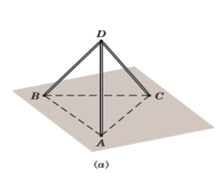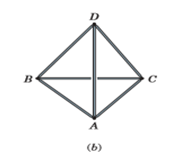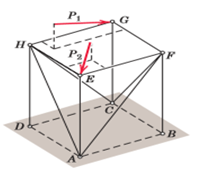Courses

# Space Trusses Mechanical Engineering Notes | EduRev

## Mechanical Engineering : Space Trusses Mechanical Engineering Notes | EduRev

The document Space Trusses Mechanical Engineering Notes | EduRev is a part of the Mechanical Engineering Course Engineering Mechanics - Notes, Videos, MCQs & PPTs.
All you need of Mechanical Engineering at this link: Mechanical Engineering

A space truss is the three-dimensional counterpart of the plane truss described in the three previous articles. The idealized space truss consists of rigid links connected at their ends by ball-and-socket joints (such a joint is illustrated in Fig. 3/8 in Art. 3/4). Whereas a triangle of pin-connected bars forms the basic noncollapsible unit for the plane truss, a space truss, on the other hand, requires six bars joined at their ends to form the edges of a tetrahedron as the basic noncollapsible unit. In Fig. 4/13a the two bars AD and BD joined at D require a third support CD to keep the triangle ADB from rotating about AB. In Fig. 4/13b the supporting base is replaced by three more bars AB, BC, and AC to form a tetrahedron not dependent on the foundation for its own rigidity.We may form a new rigid unit to extend the structure with three additional concurrent bars whose ends are attached to three ﬁxed joints on the existing structure. Thus, in Fig. 4/13c the bars AF, BF, and CF are attached to the foundation and therefore ﬁx point F in space. Likewise point H is ﬁxed in space by the bars AH, DH, and CH. The three additional bars CG, FG, and HG are attached to the three ﬁxed points C, F, and H and therefore ﬁx G in space. The ﬁxed point E is similarly created. We see now that the structure is entirely rigid. The two applied loads shown will result in forces in all of the members. A space truss formed in this way is called a simple space truss.Ideally there must be point support, such as that given by a balland-socket joint, at the connections of a space truss to prevent bending in the members. As in riveted and welded connections for plane trusses, if the centerlines of joined members intersect at a point, we can justify the assumption of two-force members under simple tension and compression.Method of joints for Space Trusses

The method of joints developed in Art. 4/3 for plane trusses may be extended directly to space trusses by satisfying the complete vector equation

ΣF = 0 (4/1)

for each joint. We normally begin the analysis at a joint where at least one known force acts and not more than three unknown forces are present. Adjacent joints on which not more than three unknown forces act may then be analyzed in turn.

This step-by-step joint technique tends to minimize the number of simultaneous equations to be solved when we must determine the forces in all members of the space truss. For this reason, although it is not readily reduced to a routine, such an approach is recommended. As an alternative procedure, however, we may simply write 3j joint equations by applying Eq. 4/1 to all joints of the space frame. The number of unknowns will be m + 6 if the structure is noncollapsible when removed from its supports and those supports provide six external reactions. If, in addition, there are no redundant members, then the number of equations (3j) equals the number of unknowns (m + 6), and the entire system of equations can be solved simultaneously for the unknowns. Because of the large number of coupled equations, a computer solution is usually required. With this latter approach, it is not necessary to begin at a joint where at least one known and no more than three unknown forces act.

Method of sections for Space Trusses

The method of sections developed in the previous article may also be applied to space trusses. The two vector equations

ΣF = 0          andΣM = 0

must be satisﬁed for any section of the truss, where the zero moment sum will hold for all moment axes. Because the two vector equations are equivalent to six scalar equations, we conclude that, in general, a section should not be passed through more than six members whose forces are unknown. The method of sections for space trusses is not widely used, however, because a moment axis can seldom be found which eliminates all but one unknown, as in the case of plane trusses. Vector notation for expressing the terms in the force and moment equations for space trusses is of considerable advantage.

Offer running on EduRev: Apply code STAYHOME200 to get INR 200 off on our premium plan EduRev Infinity!

## Engineering Mechanics - Notes, Videos, MCQs & PPTs

30 videos|72 docs|65 tests

,

,

,

,

,

,

,

,

,

,

,

,

,

,

,

,

,

,

,

,

,

;